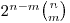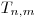# Higher dimensional Rubik's cube

#### quelramodellago

##### Member
Hi. I'm trying to define the group of the Rubik's cube in arbitrary dimension. I shall explain what i figured out till now.
Let $$T_n$$ be the group of rotational isometries of the n-dimensional cube. It acts transitively and faithfully on them-dimensional faces of the n-dimensional cube for any m between 0 and n.
Letbe the stabilizer of any of such faces (it does not depend, up to isomorphism, on the chosen face): that is, we consider the rotational isometries of the n-dimensional cube fixing a given m-dimensional face. So I define the 'illegal' n-dimensional Rubik's cube group to be:That's because each of the m-dimensional pieces of the puzzle would haveas orientation group because that piece would be 'attached' by one of its m-dimensional faces (any piece is itself an n-dimensional cube) to the 'core' of the whole puzzle.
My problem is: i can't figure out how to define the generators on that group to build the real n-dimensional Rubik's cube group. It may be useful to note that by Krasner-Kalujnin embedding we have that $$T_n$$ is embedded (in the way that we expect) in our illegal group.
Has any of you got any idea?

(sorry for the latex mess, i got problems on this forum using implemented latex tool)

#### UMichSpeedCubist

##### Member
The math is a tad beyond me, but the generators of the group is easy to explain.

Let N be the number of dimensions and for simplicity the puzzle is 3^N (as opposed to L^N, with L>3 which would have additional slice maneuvers).
Each of the 2*N facets/sides can rotate in a number of ways (not including the null-turn) -- 1 when N=2, 3 when N=3, 23 when N=4, and 191 when N=5. Pattern is (N! * 2^(N-1))-1.
However, this includes all the non-90-degree rotations (such as vertex-turns and edge-turns on a 3x3x3x3), which can be generated from a series 90-degree rotations of the same facet.
If N>2, the rotations of a facet can be generated with N-2 elements. For example, using just 2 elements you can generate all 24 rotational symmetries of a hexahedron.
Thus, the total number of generators would be 2*N*(N-2). You just gotta be careful defining those N-2 rotations of a facet in a consistent manner when N is arbitrary.

A 3x3x3 can be considered a group with 6 generators, as expected.
A 3x3x3x3 can be considered a group with 16 generators.
I believe my outline provides minimal number of generators.

-Doug

#### bcube

##### Member
Hello quelramodellago. I am sorry for a late response ( I noticed your thread 2 minutes ago).

I don't understand the math either. However, people at http://twistypuzzles.com/forum could, therefore if you still haven't found an answer to your question, I highly recommend you to ask also at that linked forum.